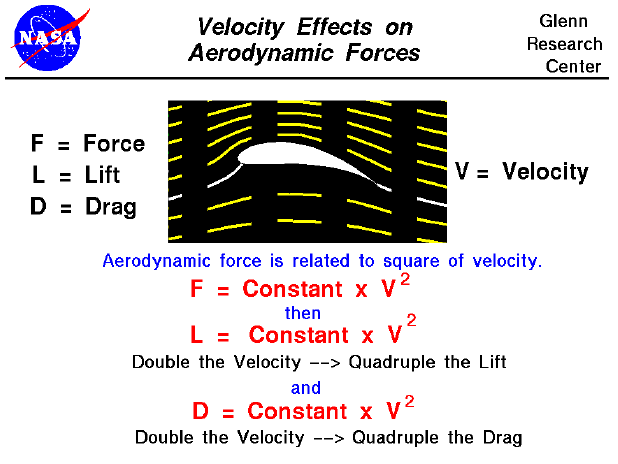Lift is created by deflecting a flow of air and drag is generated on a body in a wide variety of ways. From Newton's second law of motion, the aerodynamic forces on the body (lift and drag) are directly related to the change in momentum of the fluid with time. The fluid momentum is equal to the mass times the velocity of the fluid. Since the air moves, defining the mass gets a little tricky and aerodynamicists usually relate the effect of mass on lift and drag to the air density. The mathematical derivation for this conversion is given on another slide dealing with momentum effects on lift. As a result of this derivation, we find that lift and drag depend on the square of the velocity.

The velocity used in the lift and drag equations is the relative velocity between an object and the flow. Since the aerodynamic force depends on the square of the velocity, doubling the velocity will quadruple the lift and drag.

Let's investigate the dependence of lift on velocity using a Java simulator.

As an experiment, set the velocity to 50 mph and note the amount of lift. Now double the velocity to 100 mph. What is the value of the lift? How does it compare to the previous measurement?

You can further investigate the effect of velocity and the other factors affecting lift by using the FoilSim II Java Applet. You can also download your own copy of FoilSim to play with for free.Guided Tours

Beginner's Guide to Aerodynamics
Beginner's Guide to Propulsion
Beginner's Guide to Model Rockets
Beginner's Guide to Kites
Beginner's Guide to Aeronautics